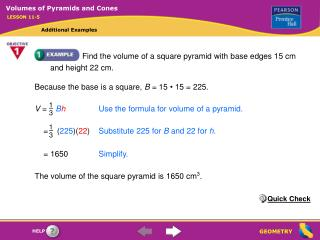DownloadDownload PresentationFind the volume of a square pyramid with base edges 15 cm and height 22 cm.

# Find the volume of a square pyramid with base edges 15 cm and height 22 cm.

Télécharger la présentation## Find the volume of a square pyramid with base edges 15 cm and height 22 cm.

- - - - - - - - - - - - - - - - - - - - - - - - - - - E N D - - - - - - - - - - - - - - - - - - - - - - - - - - -
##### Presentation Transcript

1. 1 3 1 3 V=BhUse the formula for volume of a pyramid. = (225)(22) Substitute 225 for B and 22 for h. Volumes of Pyramids and Cones LESSON 11-5 Additional Examples Find the volume of a square pyramid with base edges 15 cm and height 22 cm. Because the base is a square, B= 15 • 15 = 225. = 1650 Simplify. The volume of the square pyramid is 1650 cm3. Quick Check

2. Volumes of Pyramids and Cones LESSON 11-5 Additional Examples Find the volume of a square pyramid with base edges 16 m and slant height 17 m. The altitude of a right square pyramid intersects the base at the center of the square.

3. Because each side of the square base is 16 m, the leg of the right triangle along the base is 8 m, as shown below. Volumes of Pyramids and Cones LESSON 11-5 Additional Examples (continued) Step 1: Find the height of the pyramid. 172= 82h2Use the Pythagorean Theorem. 289 = 64 h2Simplify. 225 =h2Subtract 64 from each side. h= 15 Find the square root of each side.

4. 1 3 1 3 V=BhUse the formula for the volume of a pyramid. = (16  16)15Substitute. Volumes of Pyramids and Cones LESSON 11-5 Additional Examples (continued) Step 2: Find the volume of the pyramid. = 1280 Simplify. The volume of the square pyramid is 1280 m3. Quick Check

5. Find the volume of the cone below in terms of . 1 2 r= d = 3 in. 1 3 V=r 2hUse the formula for volume of a cone. 1 3 = (32)(11) Substitute 3 for r and 11 for h. = 33 Simplify. The volume of the cone is 33 in.3. Volumes of Pyramids and Cones LESSON 11-5 Additional Examples Quick Check

6. 1 3 d 2 V= r 2h Use the formula for the volume of a cone. r= = 2 1 3 V= (22)(7)Substitute 2 for r and 7 for h. Use a calculator. Volumes of Pyramids and Cones LESSON 11-5 Additional Examples An ice cream cone is 7 cm tall and 4 cm in diameter. About how much ice cream can fit entirely inside the cone? Find the volume to the nearest whole number. About 29 cm3 of ice cream can fit entirely inside the cone. Quick Check